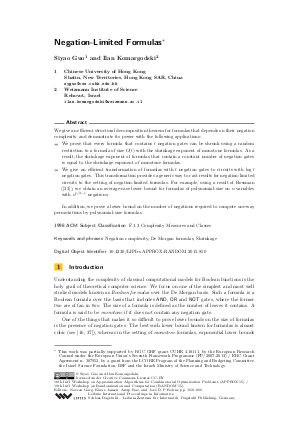Document# Negation-Limited Formulas

### Authors Siyao Guo, Ilan Komargodski## File

LIPIcs.APPROX-RANDOM.2015.850.pdf
• Filesize: 0.56 MB
• 17 pages

## Cite As

Siyao Guo and Ilan Komargodski. Negation-Limited Formulas. In Approximation, Randomization, and Combinatorial Optimization. Algorithms and Techniques (APPROX/RANDOM 2015). Leibniz International Proceedings in Informatics (LIPIcs), Volume 40, pp. 850-866, Schloss Dagstuhl - Leibniz-Zentrum für Informatik (2015)
https://doi.org/10.4230/LIPIcs.APPROX-RANDOM.2015.850

## Abstract

We give an efficient structural decomposition theorem for formulas that depends on their negation complexity and demonstrate its power with the following applications. We prove that every formula that contains t negation gates can be shrunk using a random restriction to a formula of size O(t) with the shrinkage exponent of monotone formulas. As a result, the shrinkage exponent of formulas that contain a constant number of negation gates is equal to the shrinkage exponent of monotone formulas. We give an efficient transformation of formulas with t negation gates to circuits with log(t) negation gates. This transformation provides a generic way to cast results for negation-limited circuits to the setting of negation-limited formulas. For example, using a result of Rossman (CCC'15), we obtain an average-case lower bound for formulas of polynomial-size on n variables with n^{1/2-epsilon} negations. In addition, we prove a lower bound on the number of negations required to compute one-way permutations by polynomial-size formulas.
##### Keywords
• Negation complexity
• De Morgan formulas
• Shrinkage

## Metrics

• Access Statistics
• Total Accesses (updated on a weekly basis)
0